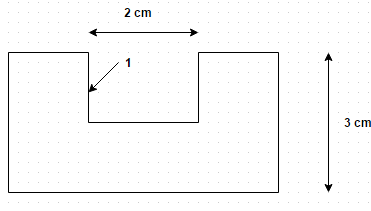## 10 Questions MCQ Test Heat Transfer for Engg. | Test: Adiabatic And Reradiating Surfaces

Description
QUESTION: 1

### Two black discs each of diameter 50 cm are placed parallel to each other concentrically at a distance of one meter. The discs are maintained at 1000 K and 500 K. Calculate the heat flow between the discs when no other surface is present

Solution:

Q = F 12 A σ (T – T 4).

QUESTION: 2

### Two black discs each of diameter 50 cm are placed parallel to each other concentrically at a distance of one meter. The discs are maintained at 1000 K and 500 K. Calculate the heat flow between the discs when the disks are connected by a cylindrical black no-flux surface

Solution:

Q = F 12 A σ (T – T 4).

QUESTION: 3

### Heat exchange between two black surfaces enclosed by an insulated surface is given by

Solution:

This is the net heat exchange between two black surfaces enclosed by an insulated surface.

QUESTION: 4

Heat exchange between two gray surfaces enclosed by an adiabatic surface is given by

Solution:

This is the net heat exchange between two gray surfaces enclosed by an adiabatic surface.

QUESTION: 5

A blind cylindrical hole of 2 cm diameter and 3 cm length is drilled into a metal slab having emissivity 0.7. If the metal slab is maintained at 650 K, make calculations for the radiation heat escape from the holeSolution:

Q = E 1 A 1 σ b T 14 [1 – F 11/1 – (1 – E 1) F 11].

QUESTION: 6

A cavity in the shape of a frustum of a cone has diameter 30 cm and 60 cm and the height is 80 cm. If the cavity is maintained at temperature of 800 K, determine the heat loss from the cavity when the smaller diameter is at the bottom

Solution:

Q = E 1 A 1 σ b T 14 [1 – F 11/1 – (1 – E 1) F 11].

QUESTION: 7

Consider the above problem, find how this heat loss would be affected if the cavity is positioned with bigger diameter at the base

Solution:

Percentage change in heat flow = 6577 – 1640/6577 = 0.7506.

QUESTION: 8

A conical cavity of base diameter 15 cm and height 20 cm has inside surface temperature 650 K. If emissivity of each surface is 0.85, determine the net radiative heat transfer from the cavity

Solution:

Q = E 1 A 1 σ b T 14 [1 – F 11/1 – (1 – E 1) F 11]. Here, F 11 = 0.649 and A 1 = 0.0503 m2.

QUESTION: 9

A cylindrical cavity of base diameter 15 cm and height 20 cm has inside surface temperature 650 K. If emissivity of each surface is 0.85, determine the net radiative heat transfer from the cavity

Solution:

Q = E 1 A 1 σ b T 14 [1 – F 11/1 – (1 – E 1) F 11]. Here, F 11 = 0.842 and A 1 = 0.11186 m2.

QUESTION: 10

What is the unit of coefficient of radiant heat transfer?

Solution:

Its value can be calculated from the heat flux equation for any configuration.